Download PresentationCryptography: Helping Number Theorists Bring Home the Bacon Since 1977

# Cryptography: Helping Number Theorists Bring Home the Bacon Since 1977 - PowerPoint PPT Presentation

Cryptography: Helping Number Theorists Bring Home the Bacon Since 1977. Dan Shumow SDE Windows Core Security dshumow@microsoft.com. Outline. Introduction Symmetric Key Encryption Key Distribution: Diffie-Hellman Key Generation Elliptic Curve Cryptography. Introduction.I am the owner, or an agent authorized to act on behalf of the owner, of the copyrighted work described.
Download Presentation## Cryptography: Helping Number Theorists Bring Home the Bacon Since 1977

An Image/Link below is provided (as is) to download presentation

Download Policy: Content on the Website is provided to you AS IS for your information and personal use and may not be sold / licensed / shared on other websites without getting consent from its author.While downloading, if for some reason you are not able to download a presentation, the publisher may have deleted the file from their server.

- - - - - - - - - - - - - - - - - - - - - - - - - - E N D - - - - - - - - - - - - - - - - - - - - - - - - - -
Presentation Transcript### Cryptography:Helping Number Theorists Bring Home the Bacon Since 1977

Dan Shumow SDE

Windows Core Security

dshumow@microsoft.com

1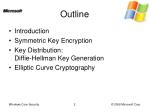Outline
• Introduction
• Symmetric Key Encryption
• Key Distribution:Diffie-Hellman Key Generation
• Elliptic Curve Cryptography

2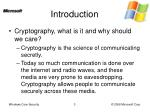Introduction
• Cryptography, what is it and why should we care?
• Cryptography is the science of communicating secretly.
• Today so much communication is done over the internet and radio waves, and these media are very prone to eavesdropping. Cryptography allows people to communicate securely across these media.

3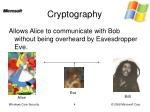Cryptography

Allows Alice to communicate with Bob without being overheard by Eavesdropper Eve.

Eve

Bob

Alice

4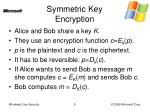Symmetric KeyEncryption
• Alice and Bob share a key K.
• They use an encryption function c=Ek(p).
• p is the plaintext and c is the ciphertext.
• It has to be reversible: p=Dk(c).
• If Alice wants to send Bob a message m she computes c = EK(m) and sends Bob c.
• Bob computes m = DK(c).

5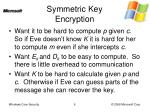Symmetric KeyEncryption
• Want it to be hard to compute p given c. So if Eve doesn’t know K it is hard for her to compute m even if she intercepts c.
• Want Ekand Dk to be easy to compute. So there is little overhead to communication
• Want K to be hard to calculate given p and c. Otherwise if Eve can guess parts of the message she can recover the key.

6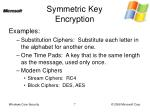Symmetric KeyEncryption

Examples:

• Substitution Ciphers: Substitute each letter in the alphabet for another one.
• One Time Pads: A key that is the same length as the message, used only once.
• Modern Ciphers
• Stream Ciphers: RC4
• Block Ciphers: DES, AES

7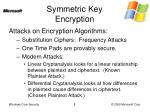Symmetric KeyEncryption

Attacks on Encryption Algorithms:

• Substitution Ciphers: Frequency Attacks
• One Time Pads are provably secure.
• Modern Attacks:
• Linear Cryptanalysis looks for a linear relationship between plaintext and ciphertext. (Known Plaintext Attack.)
• Differential Cryptanalysis looks at how differences in plaintext cause differences in ciphertext. (Chosen Plaintext Attack.)

8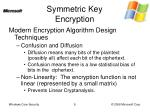Symmetric KeyEncryption

Modern Encryption Algorithm Design Techniques

• Confusion and Diffusion
• Diffusion means many bits of the plaintext (possibly all) affect each bit of the ciphertext.
• Confusion means there is a low statistical bias of bits in the ciphertext.
• Non-Linearity: The encryption function is not linear (represented by a small matrix)
• Prevents Linear Cryptanalysis.

9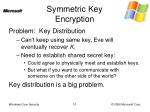Symmetric KeyEncryption

Problem: Key Distribution

• Can’t keep using same key, Eve will eventually recover K.
• Need to establish shared secret key:
• Could agree to physically meet and establish keys.
• But what if you want to communicate with someone on the other side of the world?

Key distribution is a big problem.

10Diffie-HellmanKey Generation

Basic Idea:

• Alice and Bob agree on an integer g.
• (a) Alice secretly chooses integer x, computes X = gx and sends it to Bob.(b) Bob secretly chooses integer y, computes Y = gy and sends it to Alice.
• (a) Alice computes Yx=(gy)x=gxy.(b)Bob computes Xy=(gx)y=gxy.
• Alice and Bob both share gxywhich they can use to create a secret key.

11Diffie-HellmanKey Generation

Wait!! It’s not secure. If Eve overhears what g,X, and Y are she can compute:

x = loggX and y = loggY

And use this information to calculate gxy.

To make this secure Alice and Bob pick a large prime number P and reduce everything mod P (take the remainder after division by P)

12Diffie-HellmanKey Generation

New and Improved Idea:

• Alice and Bob agree on an integer g and prime P.
• (a) Alice secretly chooses integer x, computesX = gx mod P and sends it to Bob.(b) Bob secretly chooses integer y, computes Y = gy mod P and sends it to Alice.
• (a) Alice computesYx mod P=(gy)x mod P =gxy mod P.(b)Bob computesXy mod P=(gx)y mod P =gxy mod P.
• Alice and Bob both share the value gxymod P which they can use to create a secret key.

13Diffie-HellmanKey Generation

By adding the prime P into the equation we now need to make sure that g is a “generator” of P. This means that for every integer x in {1,2,3,…,P-1}there exists an integer d such that:

x = gdmod P.

d is called the “discrete log” of g mod P.

14Diffie-HellmanKey Generation

Why Does This Work?

• Because the positive integers less than P form a multiplicative, cyclic group with generator g.
• It is hard to compute the discrete log of a generator mod P.

Given these two things:

• This algorithm works.
• It is hard for Eve to calculate gxymod P.

15Groups
• A group is a set G with a binary operation ·:G×G→Gwith the following properties:
• Associativity: a(bc)=(ab)c
• Identity Element: there exists e in G, such that for all a in Gea=ae=a.
• Inverses: for all a in G there exists an element a-1 in G such that aa-1 =a-1a = e

16Special Groups
• Abelian Groups are groups that have a fourth axiom
• Commutative: for all a and b in Gab = ba
• Cyclic Groups are groups that have a generator g. Where g is an element of G such that for all a in G:a = gxwhere x is a positive integer.Note that all Cyclic groups are Abelian.Can you see why?

17Special Groups
• Multiplicative Groups are groups where the operation is called multiplication. Example: the group of n×n invertible matrices.
• Additive Groups are groups where the operation is called addition. Additive Groups are abelian. Example: the integers.

18Diffie-Hellman Key Generation

What does this all mean for Diffie-Hellman Key Generation?

Answer: It means that Diffie-Hellman will work as a key exchange algorithm in any cyclic group where computing discrete logarithms is hard.

19Elliptic CurveCryptography
• Elliptic Curves are a way of modifying existing crypto systems like DH to make them “stronger.”
• “Stronger” means the expected time of an attack is longer with equal key sizes.
• This allows us to use smaller key sizes and therefore speed up the whole process.
• This makes ECC very useful for small devices like phones or other embedded systems.

20Elliptic Curves
• An Elliptic Curve is such an alternate cyclic group. The group consists of all points of the form: y2 = x3 + ax + b. Where x, y, a, and b are all elements of a field F.

21Fields
• A field is a set that has mathematical operations multiplication and addition that behave in nice ways.
• Basically a field is any set that you can do everything from your high school algebra class in.

22Fields

A field F is a set S along with two binary operations (+,·) that have the following properties:

• S contains two distinct elements 0 and 1
• (S-{0},·) is a multiplicative group, with identity 1.
• (S,+) is an additive group, with identity 0.
• Multiplication is distributive on the left and the right:a·(b+c) = a·b+a·c(a+b)·c = a·c+b·c

23Elliptic Curves

Group operation: Let P = (xP,yP) and Q = (xQ,yQ) be points on the an Elliptic Curve E. Then:

R = P + Q = (xR,yR)

is defined by:xR= s2-xP-xQyR=-yP+s(xP-xR)

where:s = (yP-yQ)/(xP-xQ) if xP≠xQors = (3xP2+a)/(2yP2) if xP=xQ

Identity: A “point at infinity” is added to the set of points on the curve. This point is infinitely far along the y access.

24Elliptic Curves

Intuition: If you have 2 points on this curve, they define a line that intersects the curve at 1 other point. Addition is derived from this. Inverses are reflections about the x access.

25Elliptic CurveCryptography

Newer and more Improved Idea:

• Alice and Bob agree on an Elliptic Curve E (specified by the field F and parameters a,b) and a base point g on E.
• (a) Alice secretly chooses integer x, computesX = xg and sends it to Bob.(b) Bob secretly chooses integer y, computes Y = yg and sends it to Alice.
• (a) Alice computes: xY= x(yg)=xyg.(b)Bob computes: yX= y(xg)=yxg=xyg.
• Alice and Bob both share the point xyg which they can use to create a secret key.

26Elliptic CurveCryptography
• In the preceding example all math is done in the group defined by E. Exponentiation is taken to be iterative addition.
• Because Elliptic Curves are groups we are guaranteed that we can perform all these operations.
• Computing logarithms in elliptic curves is difficult, so Eve can not recover the secret values and determine the shared value xyg.

27References
• Eric W. Weisstein. "Elliptic Curve." From MathWorld--A Wolfram Web Resource. http://mathworld.wolfram.com/EllipticCurve.html
• Eric W. Weisstein et al. "Group." From MathWorld--A Wolfram Web Resource. http://mathworld.wolfram.com/Group.html
• Eric W. Weisstein. "Field." From MathWorld--A Wolfram Web Resource. http://mathworld.wolfram.com/Field.html
• http://en.wikipedia.org/wiki/Group_%28mathematics%29
• http://en.wikipedia.org/wiki/Field_(mathematics)
• http://en.wikipedia.org/wiki/Elliptic_curves

28